Question

# An astronaut is being tested in a centrifuge. The centrifuge has a radius of 3.46 m...

An astronaut is being tested in a centrifuge. The centrifuge has a radius of 3.46 m and, in starting, rotates according to Theta = 0.30 t 2. where t is in seconds and Theta is in radians. When t = 5.81 s, what is the magnitude of the astronaut's linear speed?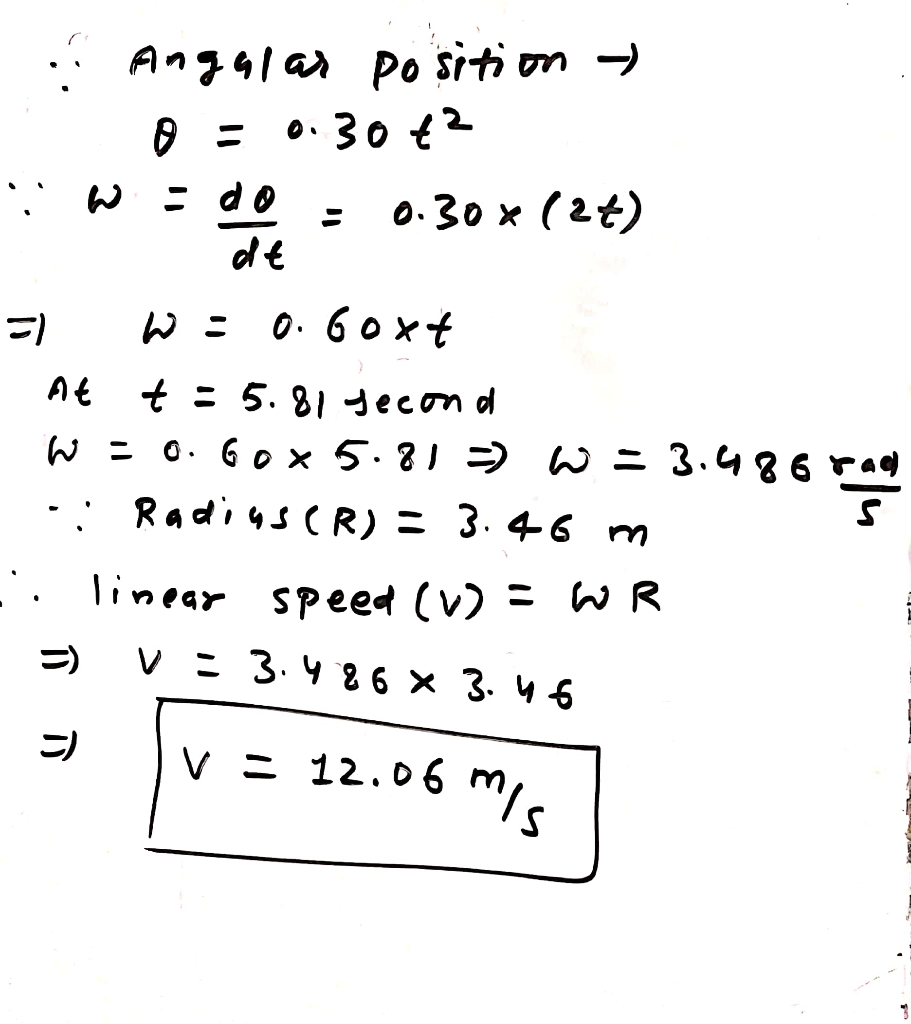#### Earn Coins

Coins can be redeemed for fabulous gifts.

Similar Homework Help Questions
• ### An astronaut is being tested in a centrifuge. The centrifuge has a radius of 9 m...

An astronaut is being tested in a centrifuge. The centrifuge has a radius of 9 m and, in starting, rotates according to θ = 0.30t2, where t is in seconds and θ is in radians. (a) When t = 11.0 s, what is the magnitude of the astronaut's angular velocity? rad/s (b) When t = 11.0 s, what is the magnitude of the astronaut's linear speed? m/s (c) When t = 11.0 s, what is the magnitude of the astronaut's...

• ### An astronaut is being tested in a centrifuge. The centrifuge has a radius of 7.40 m...

An astronaut is being tested in a centrifuge. The centrifuge has a radius of 7.40 m and, in starting, rotates according to θ = 0.190t2, where t is in seconds and θ is in radians. When t = 3.60 s, what are the magnitudes of the astronaut's (a) angular velocity, (b) linear velocity, (c) tangential acceleration, and (d) radial acceleration?

• ### An astronaut is being tested in a centrifuge. The centrifuge has a radius of 11.0 m...

An astronaut is being tested in a centrifuge. The centrifuge has a radius of 11.0 m and, in starting, rotates according to θ = 0.120t2, where t is in seconds and θ is in radians. When t = 2.90 s, what are the magnitudes of the astronaut's (a) angular velocity, (b) linear velocity, (c) tangential acceleration, and (d) radial acceleration?

• ### Current Attempt in Progress An astronaut is being tested in a centrifuge. The centrifuge has a...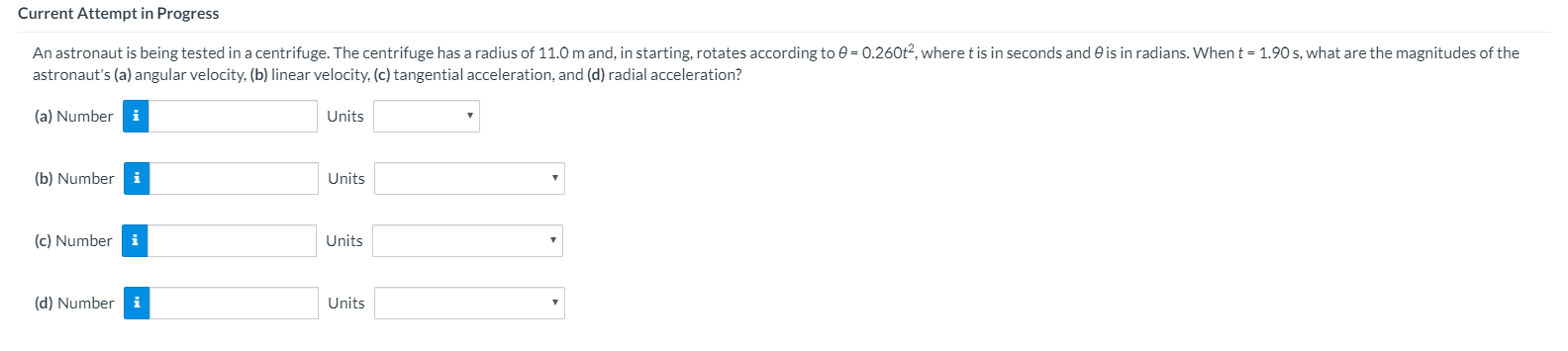Current Attempt in Progress An astronaut is being tested in a centrifuge. The centrifuge has a radius of 11.0 m and, in starting, rotates according to 0 = 0.260t?, where t is in seconds and is in radians. When t = 1.90 s, what are the magnitudes of the astronaut's (a) angular velocity, (b) linear velocity, (c) tangential acceleration, and (d) radial acceleration? (a) Number Units (b) Number i Units (c) Number i Units (d) Number Units

• ### An astronaut is being tested in a centrifuge. The centrifuge has a radius of 10 m and, in...

θ= (.30 rad/s^2)t^2, where t is in seconds and θ isin radians. When t = 5 s, what are the magnitudesof theastronaut's (a) rotational velocity, (b)translational velocity,(c) tangential acceleration, and (d) radial acceleration?I need help with getting these answers...a) 3 rad/sb) 30 m/sc) 6 m/s^2d) 90 m/s^2-Will rate lifesaver of course.

• ### Astronauts use a centrifuge to simulate the acceleration of a rocket launch. The centrifuge takes 20.0...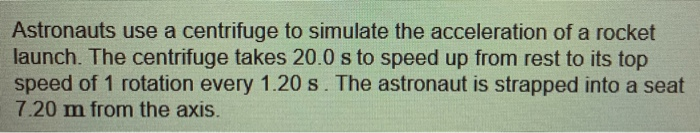Astronauts use a centrifuge to simulate the acceleration of a rocket launch. The centrifuge takes 20.0 s to speed up from rest to its top speed of 1 rotation every 1.20 s. The astronaut is strapped into a seat 7.20 m from the axis. Part A What is the astronaut's tangential acceleration during the first 20.0 s? Express your answer with the appropriate units. Value Units Submit Request Answer Part B How many g's of acceleration does the astronaut experience...

• ### Astronauts use a centrifuge to simulate the acceleration of a rocket launch. The centrifuge takes 40.0...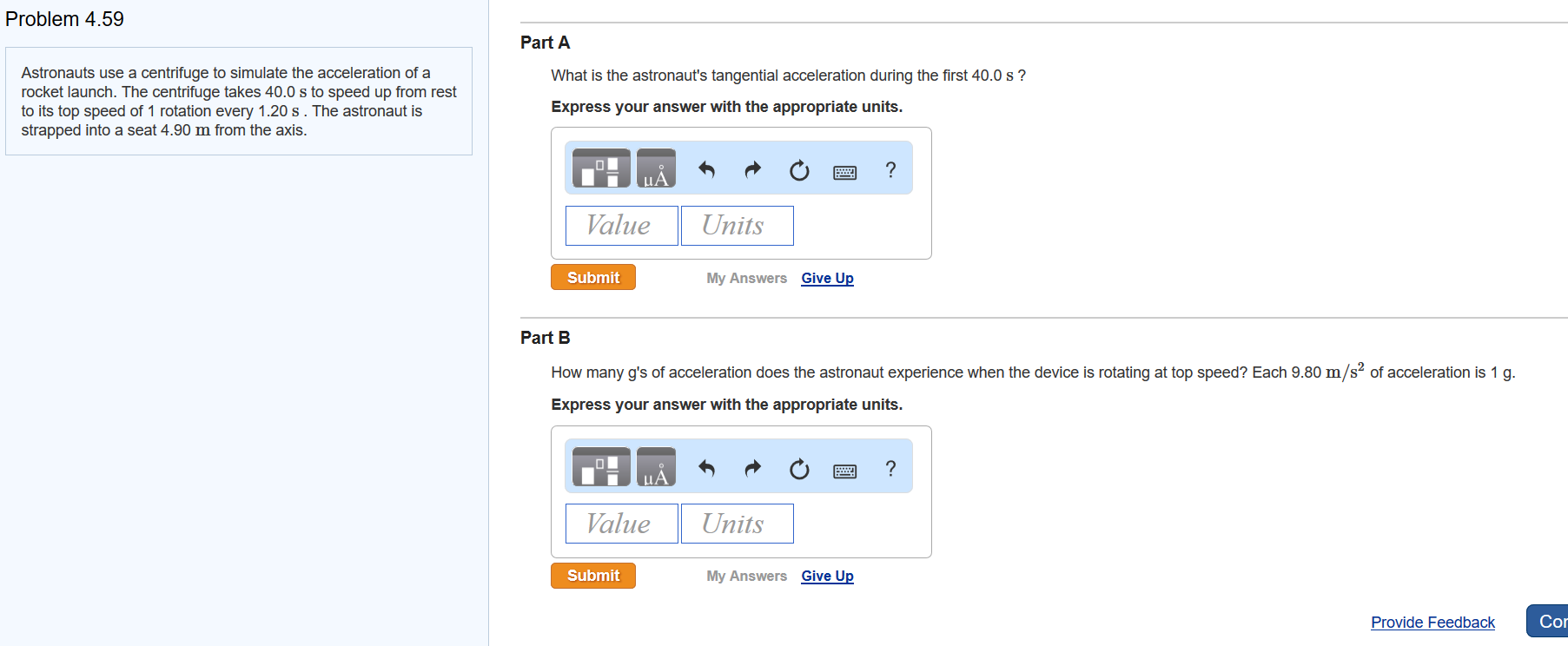Astronauts use a centrifuge to simulate the acceleration of a rocket launch. The centrifuge takes 40.0 s to speed up from rest to its top speed of 1 rotation every 1.20 s . The astronaut is strapped into a seat 4.90 m from the axis. please show work. need help with both questions. Problem 4.59 Part A Astronauts use a centrifuge to simulate the acceleration of a rocket launch. The centrifuge takes 40.0 s to speed up from rest to...

• ### Astronauts use a centrifuge to simulate the acceleration of a rocket launch. The centrifuge takes 20.0...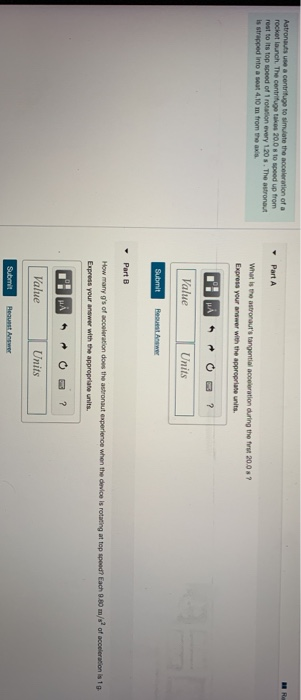Astronauts use a centrifuge to simulate the acceleration of a rocket launch. The centrifuge takes 20.0 8 to speed up from rest to its top speed of rotation every 1.20 8. The astronaut is strapped into a soat 4.10 m from the axa Part A What is the astronaut's tangential acceleration during the first 2008? Express your answer with the appropriate units. Value Units Submit Request Part B How many g's of acceleration does the astronaut experience when the device...

• ### Astronauts are spun in a device called a centrifuge as a physical test before they fly...

Astronauts are spun in a device called a centrifuge as a physical test before they fly in space. With what linear speed would an astronaut need to be spinning in order to experience an acceleration of 3 g's at a radius of 10.0 m? (1 g = 9.80 m/s^2​2​​)

• ### A 75.0 kg astronaut is training for accelerations that he will experience upon reentry. He is...

A 75.0 kg astronaut is training for accelerations that he will experience upon reentry. He is placed in a centrifuge (r = 20.0 m) and spun at a constant angular velocity of 15.0 rpm (revolutions per minute). He is then slowed and brought to a stop in 2.0 minutes. a. Find the magnitude and direction of the centripetal acceleration and force when he is spinning at constant angular velocity. b. How many g’s is the astronaut experiencing when moving at...